Molar mass of carbon dioxide

Apparatus:
-electronic scales
-carbon dioxide cylinder
-barometer, thermometer
-aluminium foil.
-1,000 mL measuring cylinder

1) Weigh a 500 mL conical flask.

2) Place some aluminium foil on the top of the flask and make a small hole in the middle as shown and weigh on an electronic scale.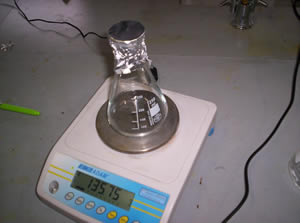3) With a carbon dioxide cylinder create some dry ice.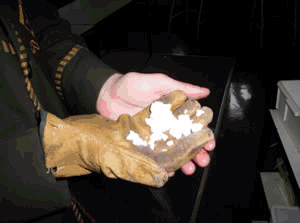4) Place about 2.00 grams into the flask and place it on the electronic scale. Observe the mass dropping as carbon dioxide escapes. Record the mass of the flask and carbon dioxide. When the mass is constant for more than 20 seconds record the final reading. You will have noticed that all the dry ice has sublimed.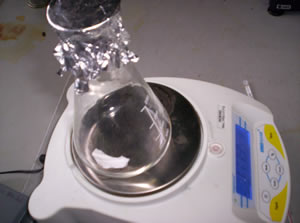4) Record the atmospheric pressure and temperature.

Convert the atmospheric pressure into kilopascals and the temperature into Kelvin.

Kelvin = Celsius + 273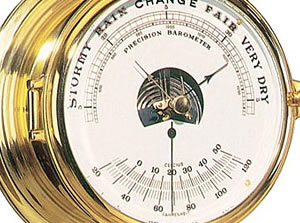5) Measure the volume of the flask by measuring the volume of water it takes to fill it to the brim.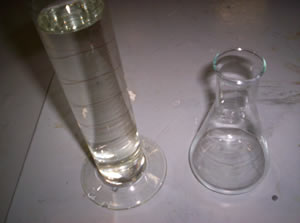6) Calculate the mass of air in the flask at room temperature using the table of the density of dry air on the right.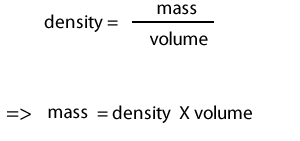T in °C ρ in kg/m3
–25 1.423
–20 1.395
–15 1.368
–10 1.342
–5 1.316
0 1.293
5 1.269
10 1.247
15 1.225
20 1.204
25 1.184
30 1.164
35 1.146

7) Calculate the mass of the glass flask.

Subtract the mass of dry air from the mass of the flask and foil as measured in 2) above.

8) Calculate the mass of carbon dioxide in the flask.

Subtract the mass of the glass flask in 7) from the mass of the flask, foil and carbon dioxide as measured in 4) above.

10) Using the formula PV = nRT calculate the formula mass in g/mol.

Transpose for M as shown on the right.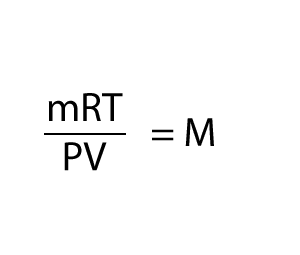1) Why should we account for the air in the flask?

2) Why does the carbon dioxide displace all the air in the flask?

3) a) Is dry air more dense than air that contains water vapour? Hint. Why do clouds float in air?

b) How would our calculation for the molar mass change if the air in the room was not dry but contained significant water vapour?

4) What would be the difference in the molar mass if the temperature was 24oC instead of 20oC?

5) Another way of producing carbon dioxide is to react an acid with a carbonate. Carbon dioxide is produced when HCl reacts with CaCO3 . A setup to recover carbon dioxide is shown on the right.

a) Why is the gas evolved in flask 1 bubbled through NaHCO3?

b) What is the composition of the gas coming from jar 1?

c) What is the composition of the gas coming from jar 2?

d) Why is the gas from jar 2 bubbled through concentrated sulfuric acid?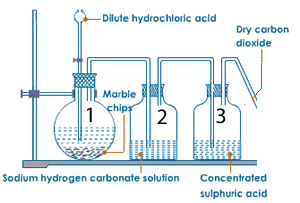Home